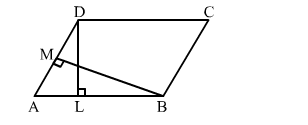# In a parallelogram ABCD, it is being given that AB = 10 cm

Question:

In a parallelogram ABCD, it is being given that AB = 10 cm and the altitudes corresponding to the sides AB and AD are DL = 6 cm and BM = 8 cm, respectively. Find AD.Solution:

ar(parallelogram ABCD) = base ​⨯ height
⇒ AB ​⨯DL = AD ​⨯ BM
⇒ 10 ​​⨯ 6 = AD ​⨯ BM
⇒ AD ​⨯ 8 = 60 cm2